# The Weird Dynamics of Viral Marketing in a Growing Market

This is the third part of a four part series of blog posts on viral marketing. In part 1, I discuss the faulty assumptions in the current models of viral marketing. In part 2, I present a better mathematical model of viral marketing. In part 4, I’ll discuss the effects of returning customers.

If the market is static, strong viral sharing can lead to rapid early growth, but once a peak is reached, the number of customers falls to zero unless your product has 100% retention. So how can a business use viral marketing to grow their customer base for the long term? Which factors (i.e. sharing rate, churn, market size, market growth) matter? In this blog post, I’ll adapt the mathematical model of viral marketing from part 2 of this series to examine how changing market size affects viral growth.

What is “the Market”:

What comprises “the market” depends on the nature of the product. If it is an iPhone game, then the market is people who own iPhones and play games on them. If it is a YouTube video of a pug climbing the stairs like a boss, then the market is people with internet connected devices who find that kind of video humorous. For the first example, entering the market could mean that you’ve bought your first iPhone, or started playing games on it. Leaving the market could mean that you’ve stopped playing games or swapped your iPhone for a different type of device. Note that leaving the market is different from becoming a former customer. In the case of an iPhone game, becoming a former customer means that you’ve stopped using the game and perhaps removed it from your phone -- you are still part of the market for iPhone games.

The Model:

If new potential customers can be added to the market and members of any subpopulation can leave the market, the parameters are now:

• $$\beta$$ - The infection rate (sharing rate)
• $\gamma$ - The recovery rate (churn rate)
• $\alpha$ -  Birth rate (rate that potential customers are entering the market)
• $\mu$ - Death rate (rate that people are leaving the market)

In part 2, I described how the population transitions from potential customers to current customers to former customers. Here, I’ll add terms to the differential equations to model how people are entering and leaving the market. Note that the total market size, $N = S + I + R$, is no longer constant, but will grow or shrink with time.

Assume that the population entering the market joins as part of the ‘potential customer’ population. The number of new potential customers joining the market per unit time is $\alpha (S + I + R)$. The populations leaving the market can come from any of the three subpopulations. The numbers of people leaving the potential customer, current customer, and former customer groups per unit time are $\mu S$, $\mu I$, and $\mu R$, respectively.

The equations become:

• $dS/dt = -\beta SI + \alpha (S + I + R) - \mu S$
• $dI/dt = \beta SI - \gamma I - \mu I$
• $dR/dt = \gamma I - \mu R$

Examining the Equations:

Since $N = S + I + R$,

$dN/dt = (\alpha - \mu )N$

which is an equation that we can solve. Thus

$N(t) = N(0) * e^{(\alpha - \mu ) t}$.

The market grows exponentially if $\alpha > \mu$ and shrinks exponentially if $\alpha < \mu$. If $\alpha = \mu$, the total market size stays the same with people entering and leaving the market at equal rates.

We can learn some more about the dynamics by examining where $dS/dt$ and $dI/dt$ are zero (that is, where the potential customer base and current customer base don't change):

$dI/dt = 0$ if $S = \frac{\gamma + \mu}{β}$ or $I = 0$ $dS/dt = 0$ if $S = \frac{\alpha N(t)}{\beta I + \mu}$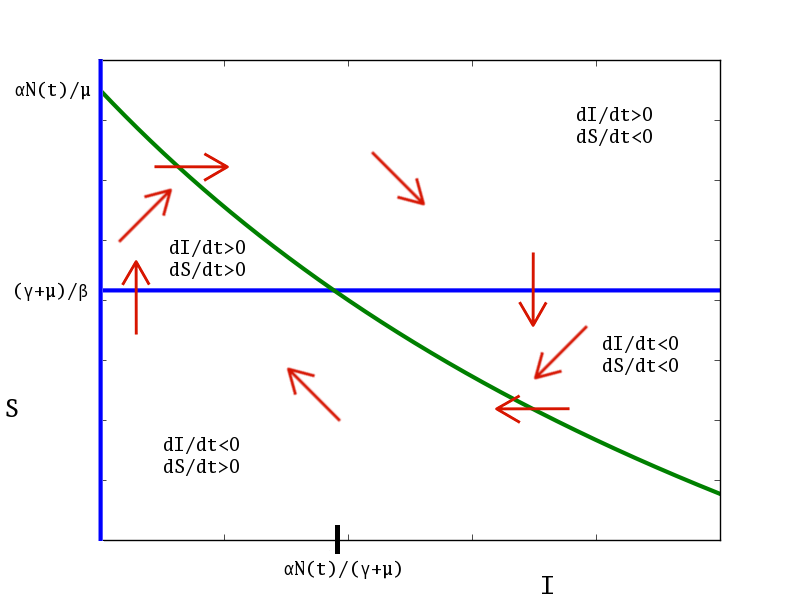Plotting these lines in the $S$ vs $I$ plane divides it into up to four regions, depending on the relative values of the parameters:

The blue lines represent where $dI/dt = 0$. The green line represents where $dS/dt = 0$. Note that since $dS/dt$ is proportional to $N(t)$, the green line will rise or lower with time depending on whether the market is growing or shrinking. The red arrows indicate the general direction the $S-I$ trajectory will be moving in while it is in each region. This suggests that if

$S(0) > \frac{\gamma + \mu}{\beta}$

then the number of number of customers grows, initially. In real terms, that means that initial growth depends on having a large enough sharing rate and number of current customers compared to the churn and "death" rates.

If

$\frac{\alpha N(0)}{\mu} > \frac{\gamma + \mu}{β}$

that is, the market size, growth rate, and sharing rate are large enough, then the number of customers may fluctuate, cyclically. In either case, the number of customers asymptotically approaches the point where both $dI/dt$ and $dS/dt$ are zero, $\frac{α}{γ + μ}N(t)$ or

$\frac{α}{γ + μ}N(0) * e^{(α - μ) t}$.

Notice that in this case, the long term behavior does not depend on the viral sharing rate, $β$!

Instead, how the number of customers grows or shrinks long term depends entirely on whether the market is growing or shrinking.

If $S(0) < \frac{γ + μ}{β}$  then the number of customers will only grow if the number of potential customers grows larger than $\frac{γ + μ}{β}$ before the number of current customers drops to zero.

In less mathematical terms, what this all means is that, if your customer base doesn't die out in the beginning, your customer base will grow exponentially, as long as you have a growing market. How fast your customer base grows in the long term depends on the growth of the market and the churn rate, but not on the viral sharing rate!

Examples:

As in Part 2, numerically integrating the differential equations allows for visualizing the effect each parameter has on viral growth.

First, compare different values of the sharing rate, β. With the parameters:

• $N(0)$ = 1 million people in the market
• $γ$ = 70% of customers lost per day
• $I(0)$ = 10 current customers
• $α$ = 14 new people in the market, per 1000 people
• $μ$ = 8 people lost from the market, per 1000 people

compare $βN(0)$ = 5, 1, 0.8, 0.5 invites per customer per day. The condition for initial growth is:

$βN(0)$

$\frac{γ + μ}{β}$

5

140,000

1

700,000

0.8

875,000

0.5

1,400,000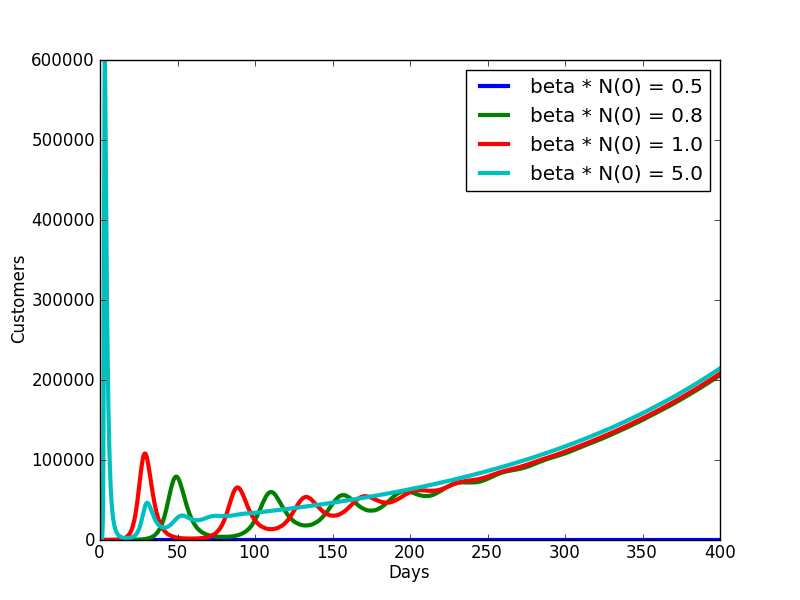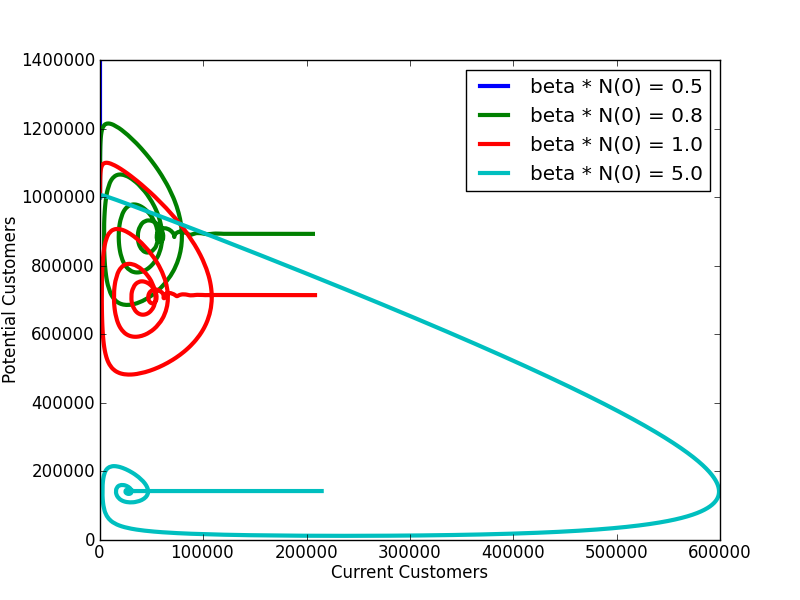For higher values of the sharing rate, $β$, a higher initial peak in the number of customers is reached and the ups and downs in the number of current customers end sooner, but the values for which the growth condition is met all follow the same pattern of growth after several months. That is, they all asymptotically approach $\frac{α}{γ + μ}N(0) * e^{(α - μ) t}$.

Now consider the effects of varying churn:

• $N(0)$ = 1 million people in the market
• $βN(0)$ = 0.8 invites per customer per day
• $I(0)$ = 10 current customers
• $α$ = 14 new people in the market, per 1000 people
• $μ$ = 8 people lost from the market, per 1000 people

compare $γ$ = 90%, 70%, 40%, or 10% of customers lost per day. The condition for growth is

$γ$

$\frac{γ + μ}{β}$

0.9

1,125,000

0.7

875,000

0.4

500,000

0.1

125,000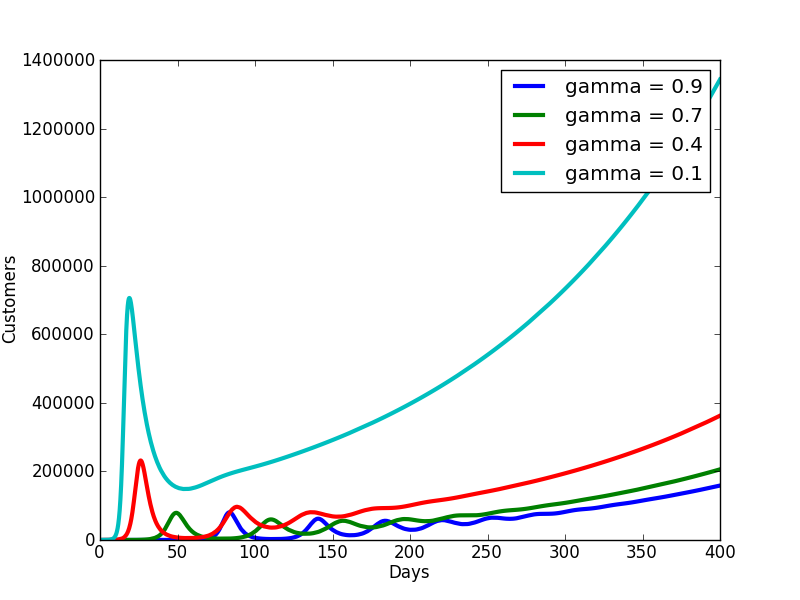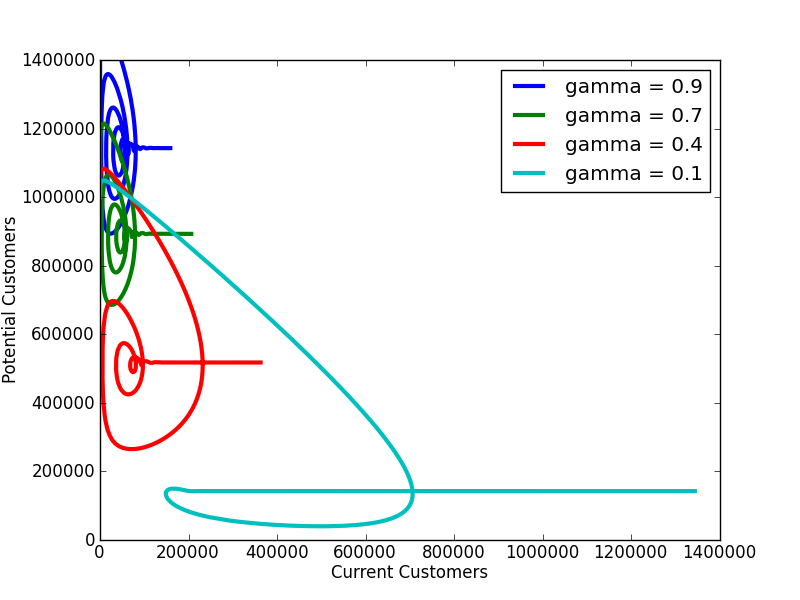Notice how the change in churn rate, $γ$, affects both the height of the initial peak and the long term growth. Lower levels of churn also lead to less of a roller coaster ride. For the case of 90% churn, the condition for growth, $S(t) > \frac{γ + μ}{β}$, is not met at $t=0$, but because of the growth of the market, $S(t)$ crosses the threshold before $I(t)$ goes to zero and viral growth is still achieved.

Finally, consider the effects of varying the growth rate of the market:

• $N(0)$ = 1 million people in the market
• $βN(0)$ = 2 invites per customer per day
• $I(0)$=10 current customers
• $γ$ = 50% customers lost per day
• $μ$ = 8 people lost from the market, per 1000 people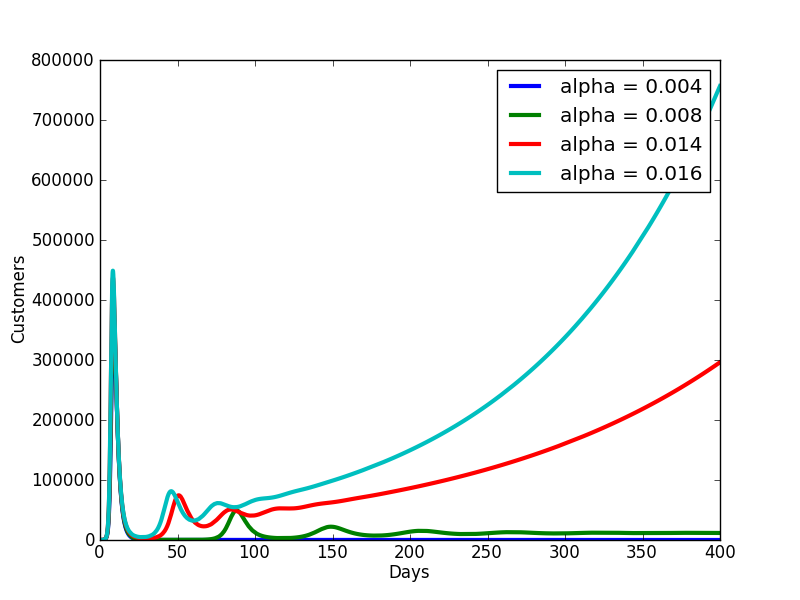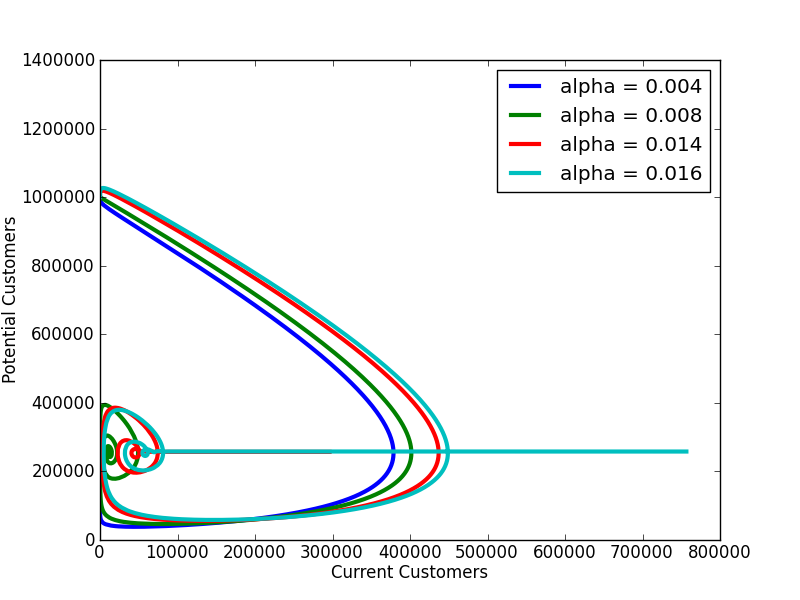compare $α$ = 4, 8, 14, 16 new people in the market, per 1000 people. The size of the initial peak is barely affected by the market growth rate, $α$, but long term behavior is affected greatly. For a “birth” rate that is lower than the “death” rate, even with strong viral sharing, the customer base drops to zero within about a month. For a $α = μ$, the customer base reaches a steady value within a year. Growing markets lead to growing customer bases, with small changes in market growth rates having a large effect in the long term.

Conclusion: All this fancy math and book learning brings us to a conclusion that is nothing new: a large and growing market is still one of the most important factors in growing a customer base for the long term. Keeping more of the customers you have also has a strong effect on long term viral growth. However the effects of a large viral sharing rate are only seen in the short term. Strong viral growth is important if your goal is to reach a large number of people quickly, as with a viral advertising campaign. But if your goal is to grow a customer base for a product for the long term, any amount of viral sharing can lead to long term growth as long as the market is growing and the churn in the customer base is low enough.

In Part 4, I'll look at the effect of returning customers.

TLDR: Differences in viral sharing rates only matter in the short term. For long term growth, the most important factors are low churn and a growing market.

Image Credit.